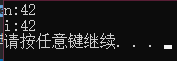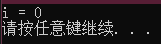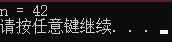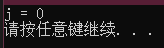# 欢迎关注WX公众号：【程序员管小亮】

## 专栏C++学习笔记

《C++ Primer》学习笔记/习题答案 总目录

——————————————————————————————————————————————————————

# C++模块设计——函数

## 1、函数基础

n的阶乘是从1到n所有数字的乘积，程序如下：

// val的阶乘是val * (val - 1) * (val - 2) . . . * ((val - (val - 1)) * 1)
int fact(int val)
{
int ret = 1;    	// 局部变量，用于保存计算结果
while (val > 1)
ret *= val--;   // 把ret和val的来积赋给ret，然后将val减1
return ret;     	// 返回结果
}


int main()
{
int j = fact(5);    // j equals 120, i.e., the result of fact(5)
cout << "5! is " << j << endl;
return 0;
}


• 用实参初始化对应的形参；
• 将控制权从主调函数转移给被调函数。

return 语句结束函数的执行过程，完成两项工作：

• 返回 return 语句中的值（如果有的话）。
• 将控制权从被调函数转移回主调函数，函数的返回值用于初始化调用表达式的结果，之后继续完成调用所在的表达式的剩余部分。

void f1() { /* ... */ }      // 隐式地定义空形参列表
void f2(void) { /* ... */ }  // 显式地定义空形参列表


int f3(int v1, v2) { /* ... */ }      // 错误
int f4(int v1, int v2) { /* ... */ }  // 正确


#### 1）局部对象

• 名字的作用域是程序文本的一部分， 名字在其中可见；
• 对象的生命周期是程序执行过程中该对象存在的一段时间。

size_t count calls() {
static size_t ctr = 0; // 调用结束后，这个值仍然有效
return ++ctr;
}
int main () {
for (size_t i = 0; i != 10; ++i)
cout << count_calls() << endl;
return 0;
}


## 2、参数传递

• 当形参是引用类型时，它对应的实参被 引用传递（passed by reference），函数被 传引用调用（called by reference）。引用形参是它对应实参的别名。
• 当形参不是引用类型时，形参和实参是两个相互独立的对象，实参的值会被拷贝给形参（值传递，passed by value），函数被 传值调用（called by value）

#### 1）传值参数

#include <iostream>
using namespace std;
int main()
{
int n = 0, i = 42;
int *p = &n, *q = &i; // p指向n; q指向i
*p = 42;			  // n的值改变; p不变
p = q;				  // p现在指向了i; 但是i和n的值都不变

cout << "n:" << n << endl;
cout << "i:" << i << endl;
system("pause");
return 0;
}// 该函数接受一个指针，然后将指针所指的位置为0
void reset(int *ip) {
*ip = 0;					// 改变指针ip所指对象的值
ip = 0;						// 只改变了ip的局部拷贝，实参未被改变
}


int i = 42;
reset(&i);						// 改变i的值而非i的地址
cout << "i = " << i << endl;	// 输出i = 0#### 2）传引用参数

#include <iostream>
using namespace std;

int main()
{
int n = 0, i = 42;
int &r = n; 		// r绑定了n(即r是n的另一个名字)
r = 42;				// 现在n的值是42
r = i;				// 现在n的值和i相同
cout << "n = " << n << endl;
system("pause");
return 0;
}// 该函数接受一个int对象的引用，然后将对象的位置为0
void reset(int &i)  			// i是传给reset函数的对象的另一个名字
{
i = 0;  					// 改变了i所引对象的值
}


int j = 42;
reset(j);						// j采用传引用方式，它的值被改变
cout << "j = " << j << endl;	// 输出j = 0#### 3）const形参和实参

void fcn(const int i) { /* fcn能够读取i，但是不能向i写值 */ }


void fcn(const int i) { /* fcn能够读取i，但是不能向i写值 */ }
void fcn(int i) { /* ... */ } 	// 错误:重复定义了fcn(int)


#### 4）数组形参

• 因为不能拷贝数组，所以无法以值传递的方式使用数组参数，但是可以把形参写成类似数组的形式。
// 尽管形式不同，但这三个print函数是等价的
// 每个函数都有一个const int*类型的形参
void print(const int*);
void print(const int[]);    // 可以看出来，函数的意图是作用于一个数组
void print(const int);  // 这里的维度表示我们期望数组含有多少元素，实际不一定

• 因为数组会被转换成指针，所以当我们传递给函数一个数组时，实际上传递的是指向数组首元素的指针。
int i = 0, j = {0, 1};
print(&i); 					// 正确：&i的类型是int*
print(j); 					// 正确: j转换成int*并指向j


• 要求数组本身包含一个结束标记；
• 传递指向数组首元素和尾后元素的指针；
• 专门定义一个表示数组大小的形参。

//正确: 形参是数组的引用，维度是类型的一部分
void print(int (&arr) ) {
for (auto elem : arr)
cout << elem << endl;
}

// &arr两端的括号必不可少:
// f(int &arr) 		// 错误:将arr声明成了引用的数组
// f(int (&arr)) 	// 正确:arr是具有10个整数的整型数组的引用


// matrix指向数组的首元素，该数组的元素是由10个整数构成的数组
void print(int (*matrix), int rowSize) { /* ... */ }

// *matrix两端的括号必不可少:
int *matrix; 		// 10个指针构成的数组
int (*matrix); 		// 指向含有10个整数的数组的指针

// 等价定义
void print(int matrix[], int rowSize) { /* ... */ }


#### 5）main：处理命令行选项

int main(int argc, char *argv[]) { /*...*/ }
int main(int argc, char **argv) { /*...*/ }

• 第一个形参 argc 表示数组中字符串的数量；
• 第二个形参 argv 是一个数组，数组元素是指向C风格字符串的指针。

Visual Studio2013 中可以设置 main 函数调试参数：#### 6）含有可变形参的函数

C++11新标准提供了两种主要方法处理实参数量不定的函数：

• 如果实参类型相同，可以使用 initializer_list 标准库类型；

• 如果实参类型不同，可以定义可变参数模板。

• C++还可以使用省略符形参传递可变数量的实参，但这种功能一般只用在与C函数交换的接口程序中。

initializer_list 是一种标准库类型，定义在头文件 initializer_list 中，表示某种特定类型的值的数组。

initializer_list 提供的操作：• vector 一样，initializer_list 也是一种模板类型，定义对象时，必须说明列表中所含元素的类型
• vector 不一样的是，initializer_list 对象中的元素永远是常量值，无法改变

void error_msg(initializer_list<string> il)
{
for (auto beg = il.begin(); beg != il.end(); ++beg)
cout << *beg << " " ;
cout << endl;
}


// expected和actual是string对象
if (expected != actual)
error_msg({"functionX", expected, actual});
else
error_msg({"functionX", "okay"});


void foo(parm_list, ...);
void foo(...);


## 3、返回类型和return语句

return 语句有两种形式，作用是终止当前正在执行的函数并返回到调用该函数的地方。

return;
return expression;


#### 1）无返回值函数

void swap (int &vl , int &v2) {
// 如果两个值是相等的，则不需要交换，直接退出
if (v1 == v2)
return;
// 如果程序执行到了这里，说明还需要继续完成某些功能
int tmp = v2;
v2 = v1;
v1 = tmp;
// 此处无须显式的return语句
}


#### 2）有返回值函数

return 语句的第二种形式提供了函数的结果。只要函数的返回类型不是 void，该函数内的每条 return 语句就必须返回一个值，并且返回值的类型必须与函数的返回类型相同，或者能隐式地转换成函数的返回类型（main 函数例外）。

• return 语句没有返回值是错误的，编译器能检测到这个错误。
• 在含有 return 语句的循环后面应该也有一条 return 语句，否则程序就是错误的，但很多编译器无法发现此错误。

// 严重错误: 这个函数试图返回局部对象的引用
const string &manip()
{
string ret;
// 以某种方式改变一下ret
if (!ret.empty())
return ret;   		// 错误：返回局部对象的引用!
else
return "Empty";		// 错误："Empty"是一个局部临时量
}


C++11规定，函数可以返回用花括号包围的值的列表。同其他返回类型一样，列表也用于初始化表示函数调用结果的临时量。如果列表为空，临时量执行值初始化；否则，返回的值由函数的返回类型决定。

• 如果函数返回内置类型，则列表内最多包含一个值，且该值所占空间不应该大于目标类型的空间。

• 如果函数返回类类型，由类本身定义初始值如何使用。

vector<string> process()
{
// . . .
// expected和actual是string对象
if (expected.empty())
return {};  								// 返回一个vector对象
else if (expected == actual)
return {"functionX", "okay"};  // 返回列表初始化的vector对象
else
return {"functionX", expected, actual};
}


main 函数的返回值可以看作是状态指示器。返回0表示执行成功，返回其他值表示执行失败，其中非0值的具体含义依机器而定。

int main()
{
if (some_failure)
return EXIT_FAILURE; // 定义在cstdlib头文件中
else
return EXIT_SUCCESS; // 定义在cstdlib头文件中
}


// 计算val!,即1 * 2 * 3 . . . * val
int factorial(int val)
{
if (val > 1)
return factorial(val-1) * val;
return 1;
}


• 循环迭代适合处理线性问题（如链表，每个节点有唯一前驱、唯一后继），
• 而递归适合处理非线性问题（如树，每个节点的前驱、后继不唯一）。

main函数不能调用它自身。

#### 3）返回数组指针

Type (*function(parameter_list))[dimension]


int (*func(int i));

// func(int i)表示调用func函数时需要一个int类型的实参
// (*func(int i))意味着可以对函数调用的结果执行解引用操作
// (*func(int i))表示解引用func的调用将得到一个大小是10的数组
// int(*func(int i))表示数组中的元素是int类型


C++11允许使用 尾置返回类型（trailing return type） 简化复杂函数声明。尾置返回类型跟在形参列表后面，并以一个 -> 符号开头。为了表示函数真正的返回类型在形参列表之后，需要在本应出现返回类型的地方添加 auto 关键字。

// func接受一个int类型的实参，返回一个指针，该指针指向含有10个整数的数组
auto func(int i) -> int(*);


int odd[] = {1,3,5,7,9};
int even[] = {0,2,4,6,8};
// 返回一个指针，该指针指向含有5个整数的数组
decltype(odd) *arrPtr(int i)
{
return (i % 2) ? &odd : &even;  // 返回一个指向数组的指针
}


## 4、函数重载

void print(const char *cp);
void print(const int *beg, const int *end);
void print (const int ia[), size_t ze);


main 函数不能重载。

Record lookup(const Account&);
bool lookup(const Account&); 	// 错误：与上一个函数相比只有返回类型不同


Record lookup(Phone);
Record lookup(const Phone);  // 重复声明了Record lookup(Phone)

Record lookup(Phone*);
Record lookup(Phone* const); // 重复声明了Record lookup(Phone*)


// 对于接受引用或指针的函数来说，对象是常量还是非常量对应的形参不同
// 定义了4个独立的重载函数
Record lookup(Account&);        // 函数作用于Account的引用
Record lookup(const Account&);  // 新函数，作用于常量引用

Record lookup(Account*);        // 新函数，作用于指向Account的指针
Record lookup(const Account*);  // 新函数，作用于指向常量的指针


const_cast 可以用于函数的重载。

// 比较两个string对象的长度，返回较短的那个引用
const string &shorterString(const string &s1, const string &s2)
{
return s1.size() <= s2.size() ? s1 : s2;
}


string &shorterString(string &s1, string &s2)
{
auto &r = shorterString(const_cast<const string&>(s1),
const_cast<const string&>(s2));
return const_cast<string&>(r);
}


• 编译器找到一个与实参 最佳匹配（best match） 的函数，并生成调用该函数的代码。
• 编译器找不到任何一个函数与实参匹配，发出 无匹配（no match） 的错误信息。
• 有一个以上的函数与实参匹配，但每一个都不是明显的最佳选择，此时编译器发出 二义性调用（ambiguous call） 的错误信息。

#### 1）重载与作用域

string read();
void print(const string &);
void print(double);     // 重载print函数
void fooBar(int ival)
{
// 不好的习惯:通常来说，在局部作用域中声明函数不是一个好的选择
void print(int);    // 新作用域：隐藏了之前的print
print("Value: ");   // 错误：print(const string &)被隐藏掉了
print(ival);    	// 正确：当前print(int)可见
print(3.14);    	// 正确：调用print(int); print(doub1e)被隐藏掉了
}


## 5、特殊用途语言特性

• 默认实参
• 内联函数
• constexpr 函数

#### 1）默认实参

typedef string::size_type sz;
string screen(sz ht = 24, sz wid = 80, char backgrnd = ' ');


// 表示高度和宽度的形参没有默认位
string screen(sz, sz, char = ' ');

string screen(sz, sz, char = '*');      // 错误：重复声明
string screen(sz = 24, sz = 80, char);  // 正确：添加默认实参


// wd、def和ht的声明必须出现在函数之外
sz wd = 80;
char def = ' ';
sz ht();
string screen(sz = ht(), sz = wd, char = def);
string window = screen();   // 调用screen(ht(), 80,' ')

void f2()
{
def = '*';      // 改变默认实参的值
sz wd = 100;    // 隐藏了外层定义的wd，但是没有改变默认值
window = screen();  // 调用screen(ht(), 80, '*')
}


#### 2）内联函数和constexpr函数

// 内联版本：寻找两个string对象中较短的那个
inline const string &
shorterString(const string &s1, const string &s2)
{
return s1.size() <= s2.size() ? s1 : s2;
}


constexpr 函数是指能用于常量表达式的函数。constexpr 函数的返回类型及所有形参的类型都得是字面值类型。

constexpr 函数的返回值可以不是一个常量。

// 如果arg是常量表达式，则scale(arg)也是常量表达式
constexpr size_t scale(size_t cnt)
{
return new_sz() * cnt;
}


scale 的实参是常量表达式时，它的返回值也是常量表达式；反之则不然：

int arr[scale(2)];  // 正确：scale(2)是常量表达式
int i = 2;          // i不是常量表达式
int a2[scale(i)];   // 错误：scale(i)不是常量表达式


constexpr 函数不一定返回常量表达式。

#### 3）调试帮助

##### 1_assert 预处理宏

assert 是一种预处理宏。所谓预处理宏其实是一个预处理变量，它的行为有点类似于内联函数。

assert (expr);


##### 2_NDE8UG 预处理变量

assert 的行为依赖于于一个名为 NDEBUG 的预处理变量的状态。

• 如果定义了 NDEBUG，则 assert 什么也不做；
• 默认状态下没有定义 NDEBUG，此时 assert 将执行运行时检查。

__func__输出当前调试的函数的名称
__FILE__存放文件名的字符串字面值
__LINE__存放当前行号的整型字面值
__TIME__存放文件编译时间的字符串字面值
__DATE__存放文件编译日期的字符串字面值

## 6、函数匹配

• 一是与被调用的函数同名；
• 二是其声明在调用点可见。

• 一是形参数量与函数调用所提供的实参数量相等；
• 二是每个实参的类型与对应的形参类型相同，或者能转换成形参的类型。

#### 1）实参类型转换

1. 精确匹配 包括以下情况：
• 实参类型和形参类型相同
• 实参从数组类型或函数类型转换成对应的指针类型
• 向实参添加顶层 const 或者从实参中删除顶层 const
2. 通过 const 转换实现的匹配
3. 通过类型提升实现的匹配
4. 通过算术类型转换或指针转换实现的匹配
5. 通过类类型转换实现的匹配

Record lookup(Account&);    	// 函数的参数是Account的引用
Record lookup(const Account&);  // 函数的参数是一个常量引用
const Account a;
Account b;
lookup(a);  					// 调用lookup(const Account&)
lookup(b);  					// 调用lookup(Account&)


## 7、函数指针

// 比较两个string对象的长度
bool lengthCompare(const string &, const string &);
// pf指向一个函数，该函数的参数是两个const string的引用，返回值是bool类型
bool (*pf)(const string &, const string &); // uninitialized


*pf 两端的括号必不可少！！！如果不写这对括号，则 pf 是一个返回值为 bool 指针的函数：

// 声明一个名为pf的函数，该函数返回bool*
bool *pf(const string &, const string &);


pf = lengthCompare; // pf指向名为lengthCompare的函数
pf = &lengthCompare; // 等价的赋位语句:取地址符是可选的

bool b1 = pf("hello", "goodbye");       		// 调用lengthCompare函数
bool b2 = (*pf)("hello", "goodbye");    		// 一个等价的调用
bool b3 = lengthCompare("hello", "goodbye");    // 另一个等价的调用


void ff(int*);
void ff(unsigned int);
void (*pf1)(unsigned int) = ff; // pf1指向ff(unsigned)

void (*pf2)(int) = ff; 		// 错误：没有任何一个ff与该形参列表匹配
double (*pf3)(int*) = ff; 	// 错误：ff和pf3的返回类型不匹配


// 第三个形参是函数类型，它会自动地转换成指向函数的指针
void useBigger(const string &s1, const string &s2, bool pf(const string &, const string &));
// 等价的声明：显式地将形参定义成指向函数的指针
void useBigger(const string &s1, const string &s2, bool (*pf)(const string &, const string &));

// 自动将函数lengthCompare转换成指向该函数的指针
useBigger(s1, s2, lengthCompare);## 参考文章

• 《C++ Primer》
12-18293508-0895
07-13846
04-141303
01-143605
03-1053万+